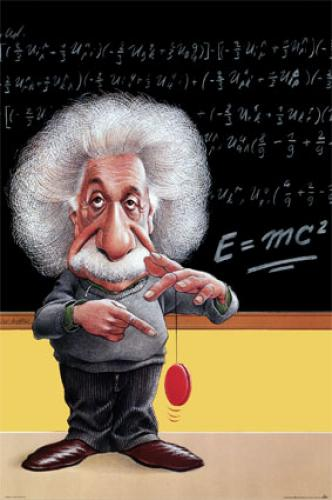## E=mc2Most people recognize this celebrated equation as something to do with Einstein’s theory of relativity, but most people have no idea what it means.

Subscribe
Notify of
Inline Feedbacks

Jew has a big nose.

Energy equals mass x the velocity of light squared.

ahh derrrr energy = mass x Velocity of light squared

most people have no idea what his theory of relativity means let alone e=mc^2.

Actually the only it has to do with relativity is that it was published the same year, 1905, as special relativity.

Technically it would be speed.

(Velocity is speed with direction)

,
You are correct about the difference between speed and velocity.
But since the speed of light is always the same regardless of frame of reference, then the velocity of light of is always equal to the speed of light.

It has to do with the amount of energy there is in an atom. That’s Einstein’s little unintentional contribution to the A-Bomb. In fact, without that, probably no one would have even thought of the A-bomb at all. Hmmmmmm…

Yeah that’s great that’s still not what it “means” thats just what the formula stands for.

What it means is: Regaurdless if an object(body)is moving it retains a certain energy as long as it has a mass.

Of course the “m” stands for relativistic mass, as opposed to normal mass. The equation is actually:

e = (m*c^3) / sqrt(c^2 – v^2)

where m is normal mass, c is the speed of light in a vacuum, and v is the velocity of the mass.

At low velocities, this reduces to e=m*c^2. As the velocity of the mass approaches the speed of light, the denominator approaches zero and the energy becomes infinite.

What then happens if you get a flashlight in space to go 99.9 percent the speed of light, then turn it on?

Will light then be going double the speed of light?

@eric, you are correct that this is the abbreviated equation and that m refers to the rest mass. That’s trivially true because of course a mass that has is not at rest also has kinetic energy. Your equation is wrong though, it has the right boundary conditions at v=0 and v=c, but but its the wrong equation. The relativistic mass is m/sqrt(1-v^2/c^2), just substitute relativistic mass into the original equation to get total energy. The difference is that the taylor series at low velocities should be approx. Newton’s 2nd law. I believe mass-energy equivalence can be derived independently from special… Read more »

@Kaze, nope, speed of light is independent to frame of reference. If you were to do your experiment, you would be experiencing time dilation. However, the photons would not be, so from your frame of reference the photons would still be traveling their usual speed. Of course, to an outside observer, the photons would be traveling the speed of light, because they see you traveling 99% of the speed of light and the photon are speeding away from you.
I haven’t had coffee yet to that explanation might be wonky.

, I worked out the taylor series for your expression and I guess it does work out for total energy. I guess in your express relativistic mass would be mc/sqrt(c^2-v^2); I guess I’ve just never seen it written out like that. Eggs on my face, serves me right for posting before coffee.

God does not play dice. he plays Texas Hold ’em.

Wow reboot, you must be a downright maniac at parties.

reboot: No problem… relativity still makes my head hurt. 8)

..and that’s just Special Reletivity.

Wait till you get to General.

Mmmm, tensors.

/not to mention QFT, where the math is so complicated you multiply pictures instead of letters

I believe your explanation is correct. The speed of light is absolute, hence there can be no accelleration beyond that. Externally, the max observed speed would be the speed of light

P.S. I thought I was bad. I’d never have voluntarily calculated the Taylor series for Erics expression. I would have just looked it up… LOL

“Scientists freeze beam of light”
news.bbc.co.uk/1/hi/sci/tech/3308109.stm